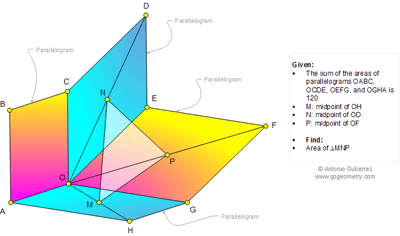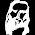## Friday, September 19, 2014

### Geometry Problem 1044: Triangle, Parallelogram, Midpoint, Area, Polya's Mind Map

Level: Mathematics Education, High School, Honors Geometry, College.

Click the figure below to view the complete problem 1044.1.Let Q is the midpoint of OB
Triangles BAH is congruent to COG and DEF ( translation transformation)
So BH=CQ=DF and BH//CQ//DF
And MQ= ½ BH, NP= 1/DF => MPNQ and BHFD are parallelograms
Note that triangle BCD is congruent to HGF and BAH is congruent to DEF
So area(MNP)= ½ Area(MPNQ)= 1/8 Area(BHFD)
= 1/8(the sum of the areas of parallelograms OABC, OCDE, OEFG, and OGHA)
=120/8= 15

2.S(BAH) = S(DEF)
S(HGF) = S(BCD)

Thus
S(BDFH) = sum of area of //gram = 120

S(MNP) = 1/4 S(HDF) = 1/8 S(BDFH) = 15

3.We denote S(XYZ...) by the area of XYZ... Connect C,E,G,A to obtain quadrilateral CEGA. Then S(CEGA)=1/2( S(OABC)+S(OCDE)+S(OEFG)+S(OGHA))=60. Let Q be the midpoint of AC. Then MPNQ is parallelogram. Since \$S(CMQ)=1/4S(CEA) and S(GPN)=1/4S(GEA) then S(CMQ)+S(GPN)=1/4S(CEGA). By the same agurment S(MEN)+S(PQA)=1/2S(CEGA). Thus S(CMQ)+S(GPN)+S(MEN)+S(PQA)=1/2S(CEGA). This implies S(MPNQ)=1/2 S(CEGA). Hence S(MNP)=1/4S(CEGA)=1/4 x 60=15.

4.S(BAH) = S(DEF)
S(HGF) = S(BCD)

120
= sum of 4 parallelograms
= S(BHFD)
= 2*S(HDF)
= 2*4*S(MNP)

S(MNP) = 15